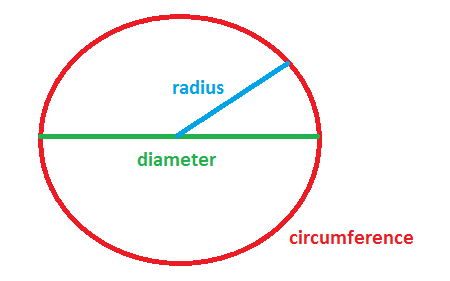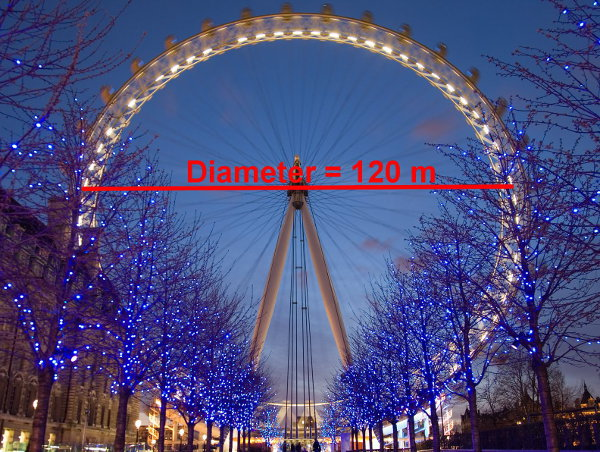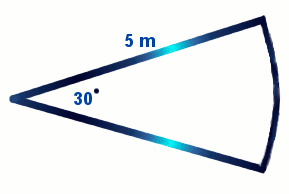# Perimeter Of Circle

In the word, "perimeter", "peri" means "around" and "meter" means "measure".

Perimeter rules are often used to work out:

• fence lengths
• picture frame lengths
• garden edging
• building trimsThe two rules for perimeter of a circle (also called the circumference) are:

Perimeter of Circle = π × D
or
Perimeter of Circle = 2 × π × r

where
π = Pi = 3.14 (Use the π button on a calculator if you can because it's more accurate.)

## Example One - Finding π

Find a round object such as a saucepan lid.
Measure the diameter.
Multiply this diameter by 3.14 (π).
Measure the circumference around the outside. Did you get the same answer?
Try this with other round objects.

## Example Two - Circumference of Circle (with Given Diameter)If the ferris wheel's diameter is 120 metres, how far would a person riding the ferris wheel travel in one rotation? (Use π = 3.14.)

Answer:
Perimeter/Circumference of circle
= π × D
= 3.14 × 120
= 376.8 metres

## Example Three - Circumference of Circle (with Given Radius)If the Earth's radius is 6371 metres, what is its circumference? (Use π = 3.14.)

Answer:
Perimeter/Circumference of circle
= 2 × π × r
= 2 × 3.14 × 6371
= 40 009.88 kilometres

## Maths Fun - How Fast Do You Spin?

At the Equator (the widest part of the Earth), the radius of the Earth from the centre to the surface is 6371 km. This means that the circumference of the Earth at the Equator is about 40000 km. Each day, the Earth spins once on its axis. This means that the Earth spins the circumference of 40000 km in 24 hours. If you lived on the Equator, how fast would you be spinning?

## Example Four - Semicircular Awning TrimThe diameter across this semicircular awning is 4 metres. How long is the green semicircular trim around the awning? (Use π = 3.14.)

Answer:
0.5 × Perimeter of circle
= 0.5 × π × D
= 0.5 × 3.14 × 4
= 6.28 m

## Example Five - Perimeter of SectorCalculate the perimeter around this sector. (Use π = 3.14.)

Answer:
Perimeter of sector
= (30° ÷ 360°) × (2 × π × r) + (2 × r)
= (30° ÷ 360°) × (2 × 3.14 × 5) + (2 × 5)
= 12.62 m

## Questions

(Use the π button on your calculator. Round your answers to 2 decimal places.)

Q1. What is the length of garden edging around a circular garden with a diameter of 20 metres?

Q2. How long is the frame around a circular portrait painting whose radius is 16 cm?

Q3. What is the whole perimeter of a sector with an angle of 120 degrees and a radius of 12 cm?

Answers
A1. 62.83 m
A2. 100.53 cm
A3. 49.13 cm

## Maths Fun - Mars RoverOpportunity, the robotic Mars Rover which was launched in 2004 and finally stopped working in 2010, travelled 34 kilometres in 90 sol (where 1 sol = 1 martian Day = 24 h 39 minutes). If the diameter of each of its wheels is approximately 15 cm, how many turns did each wheel turn in this time? What was its average speed?

## Did You Know That...?

While sitting in your chair, lift your right foot off the floor and make clockwise circles with it. Now, while doing this, draw the number 6 in the air with your right hand. Does your foot change direction? Did your hand outsmart your foot?